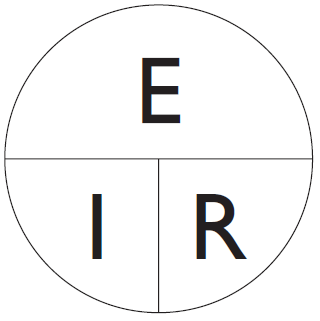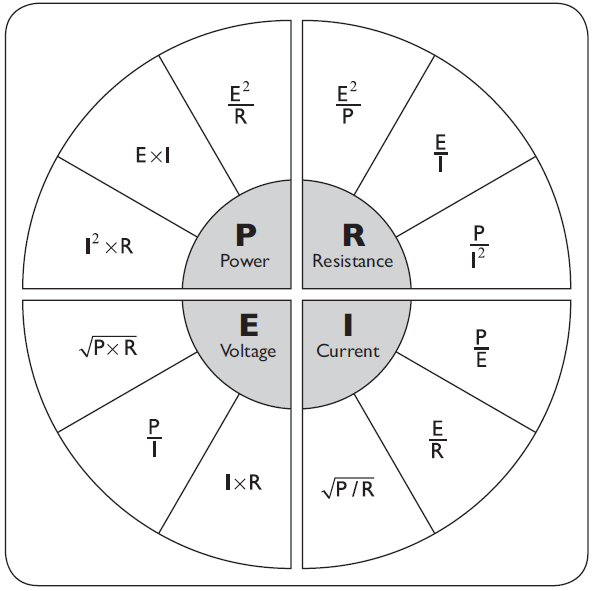### Ohm’s Law Formula

Ohm's Law
Voltage = Current × Resistance
Current = Voltage ÷ Resistance
Resistance = Voltage ÷ CurrentFig. 1  Ohm’s Law diagram

E ÷ I = R
E ÷ R = I
I × R = E

WattsFig. 2  Watt’s Law circle

P ÷ E = I
P ÷ I = E
I × E = P

12 formulas can be formed by combining Ohm’s Law and Watt’s LawFig. 3  The 12 Watt’s Law formulas